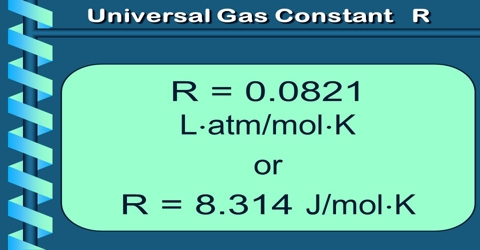# Numerical Value of Universal Gas Constant (R)

Numerical Value of Universal Gas Constant (R)

The numerical value of R may be calculated by determining the volume occupied by 1 mole of a gas at a given pressure and a given temperature. The dimension of R may be easily deduced from the equation: PV = RT

so, R = (PxV mol-1)/T

= (force/area) x (volume mol-1/temperature)

= (force/length2) x (length3.mol-1/ temperature)

= force x length x (mol-1/temperature)

= [(work x mol-1)/ temperature]; (here energy mol-1K-1)

The gas constant R is 8.314 J/mol. K. Convert the numerical value of R so that its units are cal / (mol. K).

Thus, the product PV has the dimension of work, and R may be expressed in any energy unit per mole per degree Kelvin. The constant R appears in many formulas in chemistry. The numerical value of R to be used depends on the system of units employed in the equation under consideration.

(a) Litre-atmosphere units: In equation it was pointed out that the molar volume at S.T.P., at 1 atmosphere pressure and 0°C or 273.16`K is 22.414 L. If these values are substituted in equation PV = RT; one obtains,

R = (1 x 22.414)/273.16 = 0.0820 L atm K-1 mol-1

(b) C.G.S. units: In these units pressure is expressed in dynes per square centimeter and volume in cubic centimeters.

P= 1 atm = 76 cm of Hg

=76 x13.6 x 981 dynes cm-2

V = 22.414 L = 22.414 cm3 mol-1.

R = [(76 x 13.6 x 981 x 22.414)/27316] = 8.316×107 ergs K-1mol-1